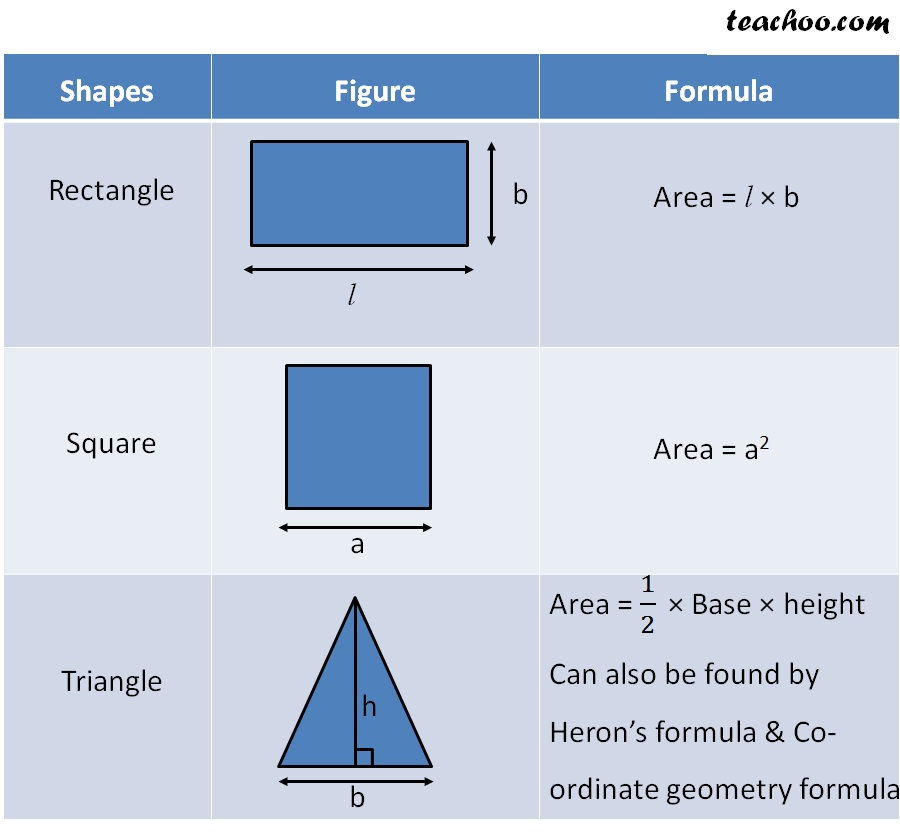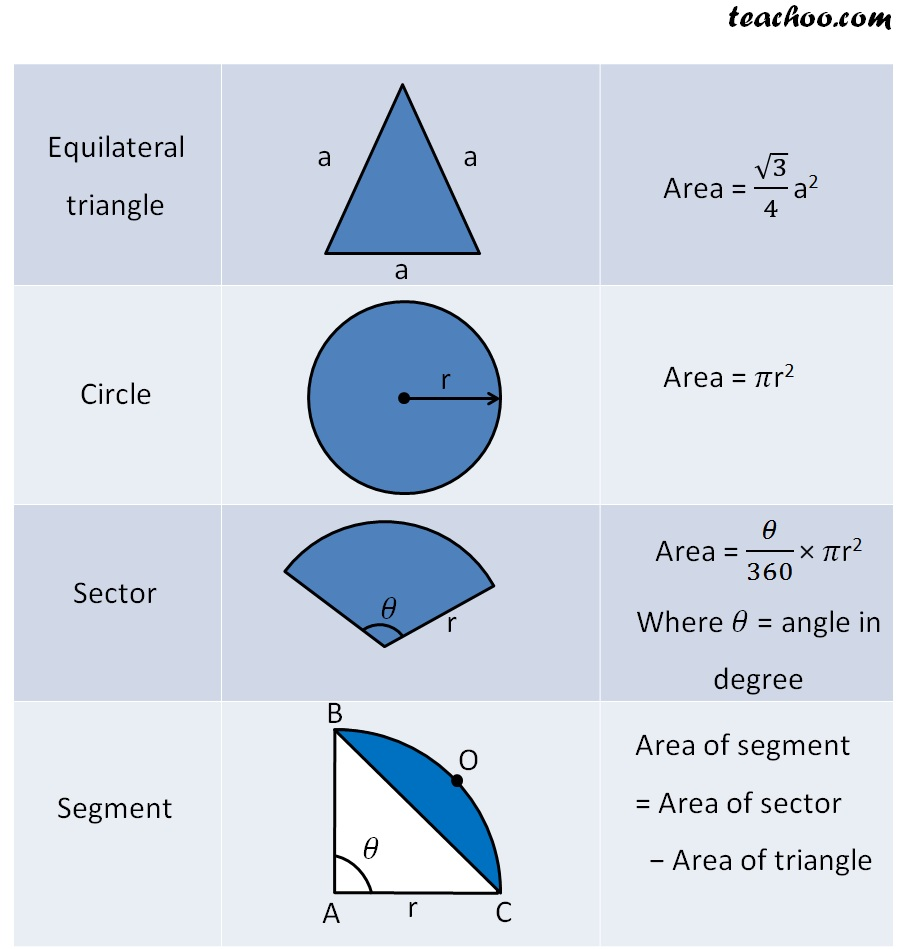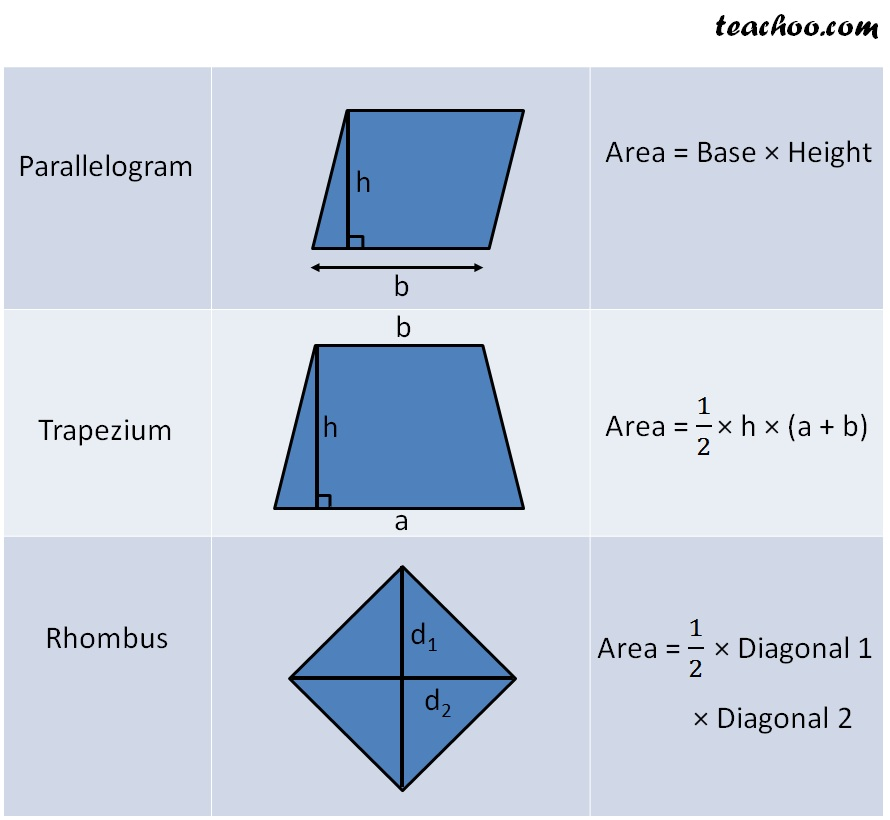Area and Perimeter

Chapter 9 Class 8 Mensuration
Concept wiseLearn in your speed, with individual attention - Teachoo Maths 1-on-1 Class

### Transcript

Shapes Figure Formula Rectangle Area = l × b Square Area = a2 Triangle Area = 1/2 × Base × height Can also be found by Heron’s formula & Co- ordinate geometry formula Equilateral triangle Area = √3/4 a2 Circle Area = 𝜋r2 Sector Area = 𝜃/360 × 𝜋r2 Where 𝜃 = angle in degree Segment Area of segment = Area of sector − Area of triangle Parallelogram Area = Base × Height Trapezium Area = 1/2 × h × (a + b) Rhombus Area = 1/2 × Diagonal 1 × Diagonal 2